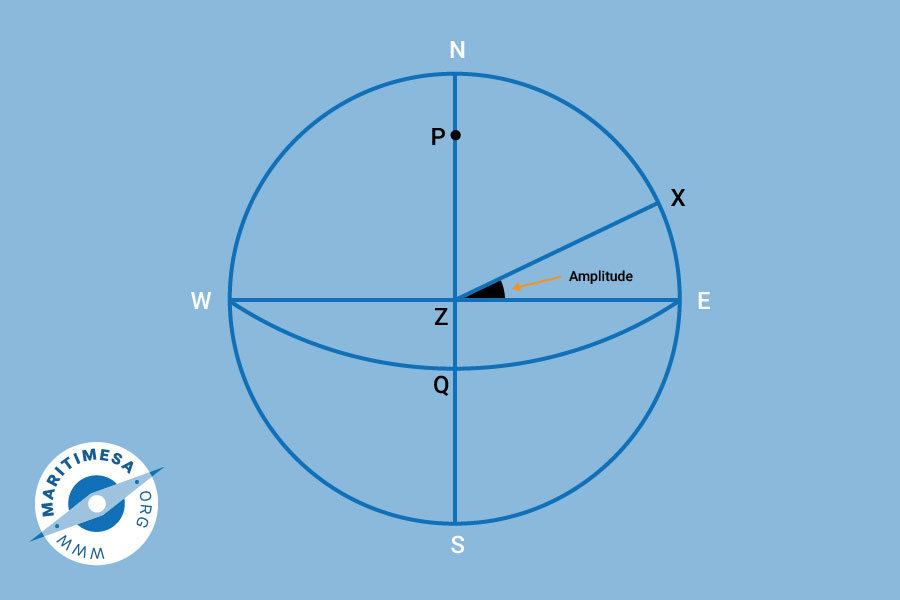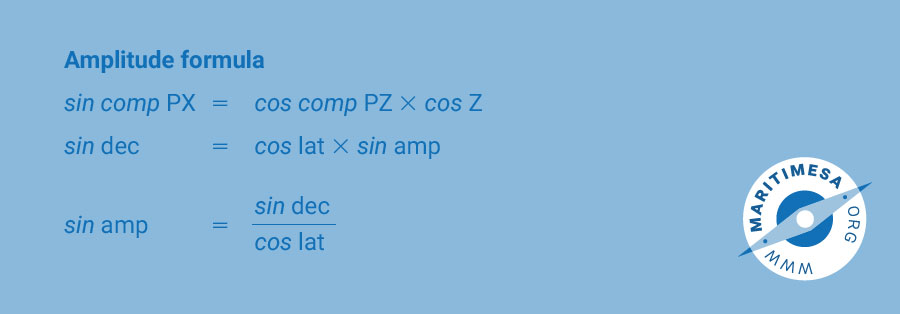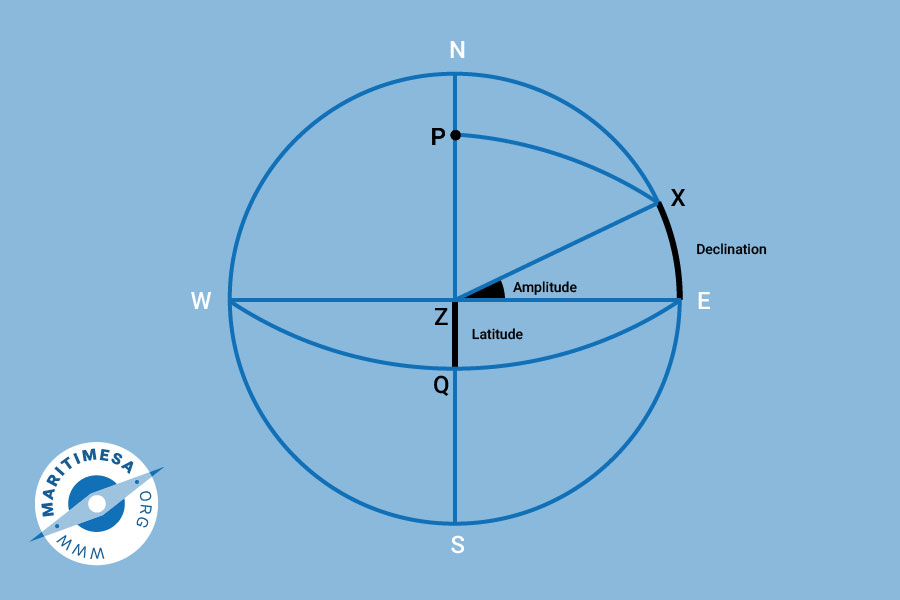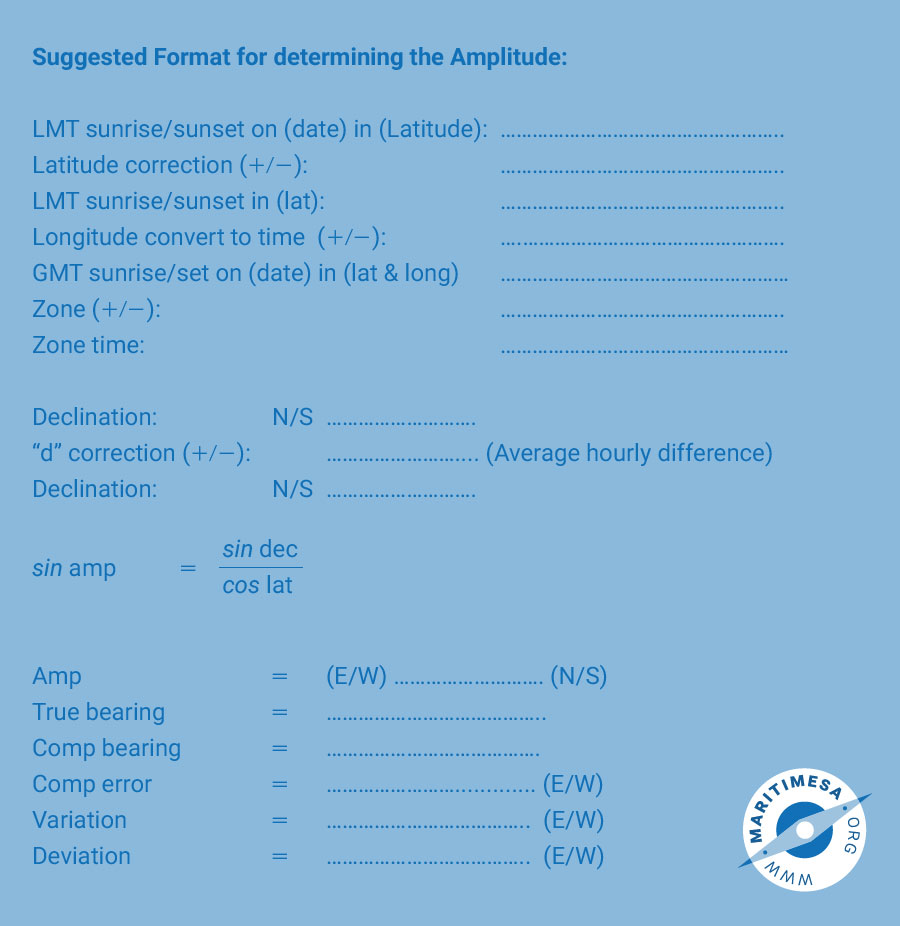# The amplitude

An amplitude is the “azimuth” of a heavenly body when it is on the observer’s rational horizon. The angle at the zenith between the prime vertical circle (the vertical circle passing through the east and west points) and the vertical circle passing through the body is called the amplitude of the body.An amplitude is the “azimuth” of a heavenly body when it is on the observer’s rational horizon.

Amplitude formula

To find the amplitude, a simple formula for the solution of the PZX triangle formed by the pole, the zenith and the body, is used. At the rising and the setting of the body, the zenith distance is 90°.
According to Napier’s rules:Procedure to determine compass error through use of amplitude:

1. Observe the compass bearing of the body at the time of theoretical rising or setting. The GMT of the occurrence may be worked out before hand.
2. Extract the declination from the nautical almanac.
3. With a DR position solve the amp formula.
4. Compare the true bearing with the compass bearing to find the compass error and if necessary, the deviation.Notes:

1. The amplitude is named the same as the declination and E or W depending on whether the sun is rising or setting.
2. The compass error is determined and named as follows:
• It is the difference between the compass and the true bearing.
• It is named E (east) if the true bearing is greater than the compass bearing.
• It is named W (west) if the true bearing is less than the compass bearing.
3. The deviation is derived and named as follows:
• If the variation and the compass error are named the same then the deviation is the numerical difference between the two,ie the smaller is subtracted from the larger.
• If the compass error is smaller than the variation then the compass error is named the opposite to the variation. If the compass error is greater than the variation then the compass error is named the same as the variation.
• If the compass error and the variation are named opposite to one another, then the deviation is the numerical sum of the two. This event is extremely unlikely since it would result in a deviation greater than the variation. In a test or exam this would indicate an error in the question being set.